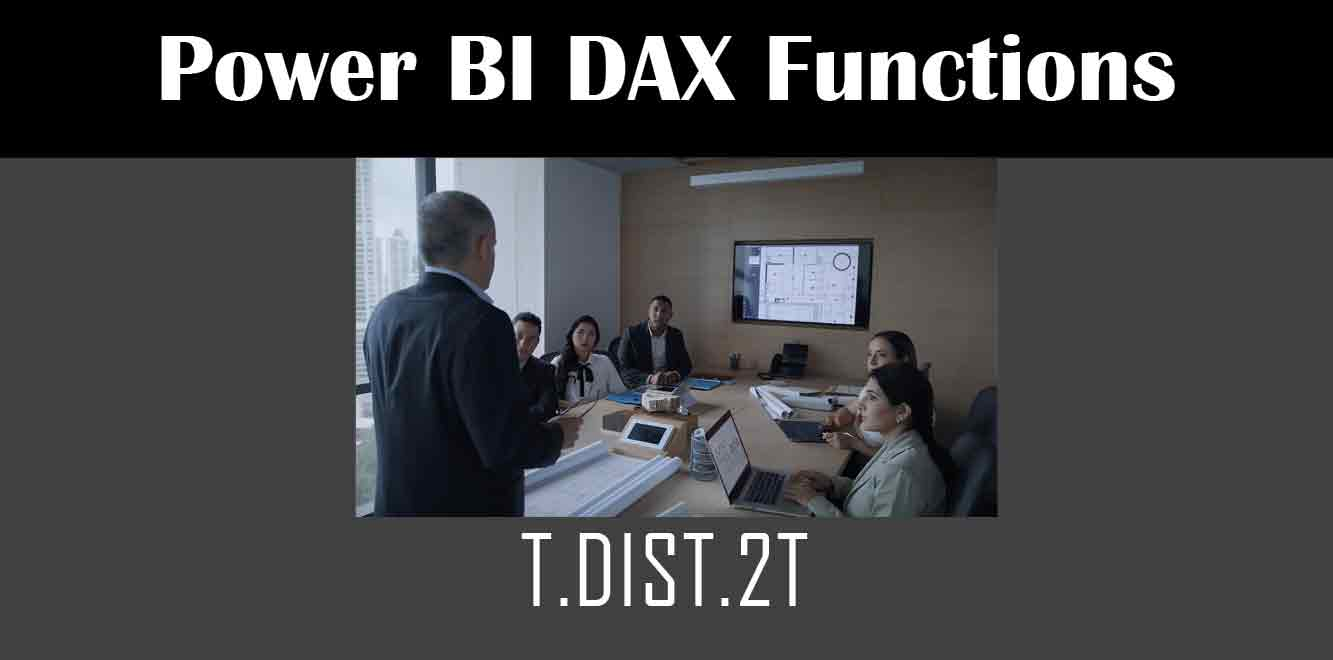# T.DIST.2T

## YOne of the functions available in DAX is T.DIST.2T. This function calculates the two-tailed probability of the Student’s t-distribution. In this article, we will explore how to use the T.DIST.2T function in Power BI.

## Syntax of T.DIST.2T Function

The syntax of the T.DIST.2T function is as follows:

T.DIST.2T(x, degrees_freedom)

``` The `x` parameter is the numeric value at which to evaluate the distribution. The `degrees_freedom` parameter is the number of degrees of freedom. Example Calculation Suppose we have a dataset that contains the following information: | Student | Test Score | |---------|------------| | Alice | 85 | | Bob | 72 | | Charlie | 91 | | Dave | 78 | | Ellen | 89 | We want to calculate the two-tailed probability of the t-distribution for a test score of 80 with 4 degrees of freedom using the T.DIST.2T function. The calculation for this would be: ```

T.DIST.2T(ABS((80 – AVERAGE(Test Score)) / (STDEV.P(Test Score) / SQRT(COUNT(Test Score)))), 4)

``` The `ABS` function returns the absolute value of the difference between the test score and the average test score. The `AVERAGE` function calculates the average test score. The `STDEV.P` function calculates the standard deviation of the test scores. The `SQRT` function calculates the square root of the number of test scores. Using T.DIST.2T Function in Power BI To use the T.DIST.2T function in Power BI, follow these steps: 1. Open Power BI Desktop and create a new report. 2. Import your data into Power BI. 3. Go to the "Modeling" tab. 4. Click on "New Measure". 5. In the formula bar, type the syntax for the T.DIST.2T function, replacing the `x` and `degrees_freedom` parameters with your own values. 6. Press "Enter" to create the measure. Your T.DIST.2T function is now ready to be used in your report. The T.DIST.2T function is a valuable tool for calculating the two-tailed probability of the Student's t-distribution in Power BI. By following the steps outlined in this article, you can easily use this function in your own reports and gain valuable insights into your data. Power BI DAX Training Courses by G Com Solutions (0800 998 9248)Power BI DAX Intensive Training Course £1,050.00 – £26,550.00 Select optionsContinue Loading DonePower BI DAX Introduction £395.00 – £9,750.00 Select optionsContinue Loading DonePower BI DAX Intermediate £395.00 – £9,750.00 Select optionsContinue Loading DonePower BI DAX Advanced £395.00 – £9,750.00 Select optionsContinue Loading Done Upcoming Courses Contact Us Subject Your Name (required) Company/Organisation Email (required) Telephone Training Course(s) Power BI Intensive TrainingPower BI introduction Power BI IntermediatePower BI AdvancedDAXPower Query MPower BI CertificationPower BI AdministrationPower PlatformPower AutomatePower AppsOTHER Your Message Upload Example Document(s) (Zip multiple files) ```
``` ```
``` ```
``` ```
``` ```
``` ```